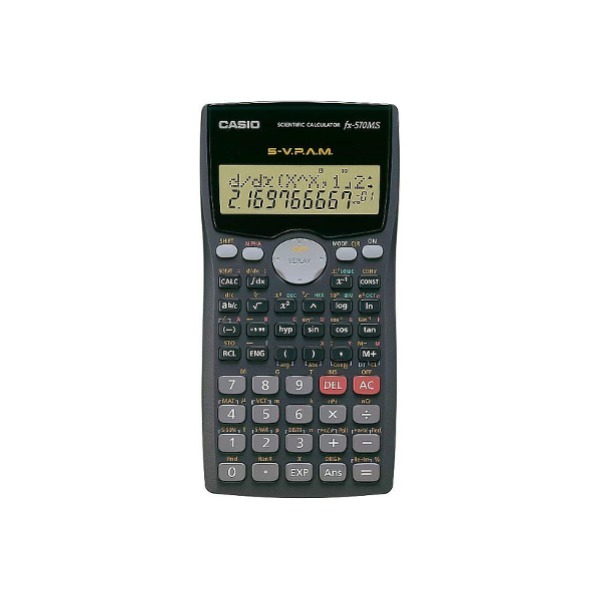# CASIO FX570MS SCIENTIFIC CALCULATOR

RM 83.00
• 10 digit mantissa + 2 digit exponential display
• Dot matrix 2-line display
• Regular percentage
• Multi-replay CALC memory
• Logical operations
• Engineering symbol calculations
• S-V.P.A.M. (Super Visually Perfect Algebraic Method)
• Fraction calculations
• Combination and permutation
• Statistics (STAT-data editor, Standard deviation, Regression analysis)

### fx-82MS/85MS/350MS functions, in addition to:

• Equation calculations
• Integration/differential calculations
• Base-n calculations/conversions
• Complex number calculations
• Matrix calculations
• Vector calculations
• 40 scientific constants

• 9 variable memories
• Comes with slide-on hard case
• Plastic keys
• Dimensions (D × W × H) : 155×78×13mm
• Weight : 105g